# Aalen-Johansen Estimator

## Definition

The Aalen-Johansen estimator is a multi-state (matrix) version of the Kaplan–Meier estimator for the hazard of a survival process. The estimator can be used to estimate the transition probability matrix of a Markov process with a finite number of states. 

## Estimator

The position in state space for an entity$i$ in continuous time$t$ is a random variable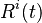$R^i(t)$ taking values in the state space S (We assume a finite state space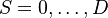$S ={0, \dots ,D}$).

The estimator is given by the expression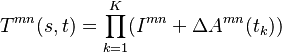$T^{mn}(s,t) = \prod_{k=1}^{K} (I^{mn} + \Delta A^{mn}(t_k))$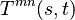$T^{mn}(s, t)$ is the transition matrix element from time s to time t, the mn-th element of the matrix denotes the probability that the Markov process starting in state m at time s will be in state n at time t. The summation is over all times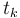$t_k$ where transition events are observed (a total of K).

### Off-diagonal elements

The estimation of the transition intensities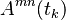$A^{mn}(t_k)$ at any time$t_k$ where transitions are observed is simply by counting: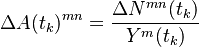$\Delta A(t_k)^{mn} = \frac{\Delta N^{mn}(t_k)}{Y^{m}(t_k)}$

where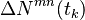$\Delta N^{mn}(t_k)$ is the number of transitions observed from state m to state n at time$t_k$ and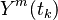$Y^m(t_k )$ is the number of entities in state m right before time$t_k$

### Diagonal elements

The diagonal elements are given by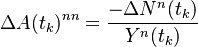$\Delta A(t_k)^{nn} = \frac{- \Delta N^{n}(t_k)}{Y^{n}(t_k)}$

where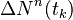$\Delta N^{n}(t_k)$ is the number of transitions away from state n at time$t_k$